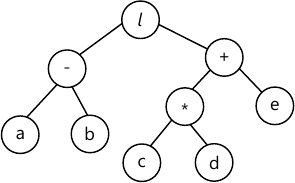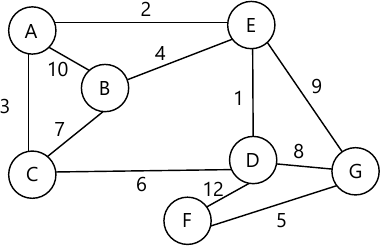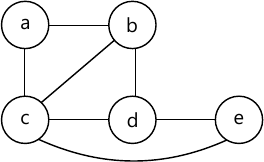MORE IN Data Structures & Algorithms
SPPU Electronics and Telecom Engineering (Semester 3)
Data Structures & Algorithms
May 2014
Total marks: --
Total time: --
INSTRUCTIONS
(1) Assume appropriate data and state your reasons
(2) Marks are given to the right of every question
(3) Draw neat diagrams wherever necessary

Answer any one question from Q1 and Q2
1 (a) Sort the following numbers using Bubble Sort. Show all steps and discuss the time complexity. 20 5 18 7 21 6
6 M
1 (b) Explain the term Data Structure and its operations.
6 M

2 (a) What is a String? Explain the usage of string functions strcmp and strlen.
6 M
2 (b) Explain in detail: Index Sequential Search and Local and Global Variables.
6 M

Answer any one question from Q3 and Q4
3 (a) Write an algorithm with appropriate illustrations to perform the following operations on Singly Linked list (SLL). Delete a node (Start, end, intermediate).
6 M
3 (b) What is the disadvantage of Linear Queue? Suggest suitable method to overcome.
6 M

Answer any one question from Q4 and Q5
4 (a) Complete following missing expression in the table.
 Infix Prefix Postfix (A+B*C)/(D+E/F) - - - +A*BC - - - ABC+*EF/
6 M
4 (b) What is a Doubly Linked List? Compare it with Singly Linked List in terms of pros and cons.
6 M

Answer any one question from Q5 and Q6
5 (a) Using the following data, draw a Binary Search Tree. Show all steps. 10 60 40 28 14 50 5
4 M
5 (b) What is a AVL Tree ? Explain with a suitable example RR and LL rotation.
4 M
5 (c) Define the following terms with respect to Trees:
i) Root
ii) Subtree
iii) Level of node
iv) Depth of Tree
v) Sibling
5 M

6 (a) Define Binary Tree. What are its types? Explain with suitable figures.
3 M
6 (b) Write a C function to search element in binary search tree.
4 M
6 (c) Write inorder, preorder and postorder traversals for the following tree.6 M

Answer any one question from Q7 and Q8
7 (a) Write an algorithm for BFS Traversal of Graph.
4 M
7 (b) Write an algorithm to find in-degree and out-degree of a vertex with a suitable example.
4 M
7 (c) Write Kruskal's algorithm for the given graph hence find minimum spanning tree.5 M

8 (a) What is a minimum spanning tree? Explain with suitable example Prism algorithm.
4 M
8 (b) Represent the following graph using adjacency matrix and adjacency list.5 M
8 (c) Explain the term topological sorting a suitable example.
4 M

More question papers from Data Structures & Algorithms